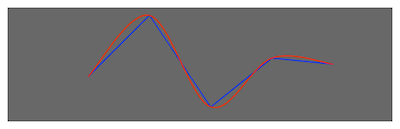# Creating a Shape Node from an Array of Points

Create jagged or smooth shapes from the same array of points.

### Overview

An `SKShapeNode` object can be initialized with an array of points describing a path. The `init(splinePoints:count:)` method can smoothly interpolate between these points to create a curve rather than the series of straight lines created by `init(points:count:)`. The following code shows how to create two shape nodes using the same array of points for both initializers.

``````var points = [CGPoint(x: 0, y: 0),
CGPoint(x: 100, y: 100),
CGPoint(x: 200, y: -50),
CGPoint(x: 300, y: 30),
CGPoint(x: 400, y: 20)]
let linearShapeNode = SKShapeNode(points: &points,
count: points.count)
let splineShapeNode = SKShapeNode(splinePoints: &points,
count: points.count)
``````

The following image shows linearShapeNode in blue and splineShapeNode in red.